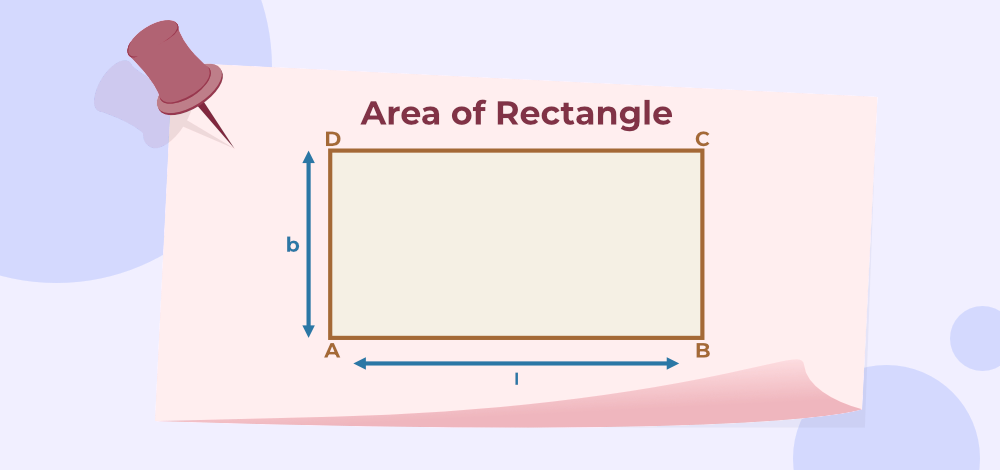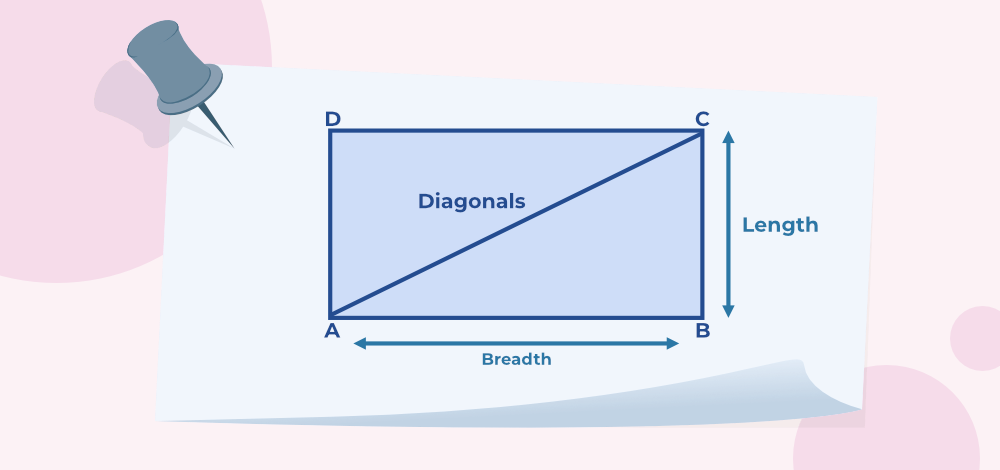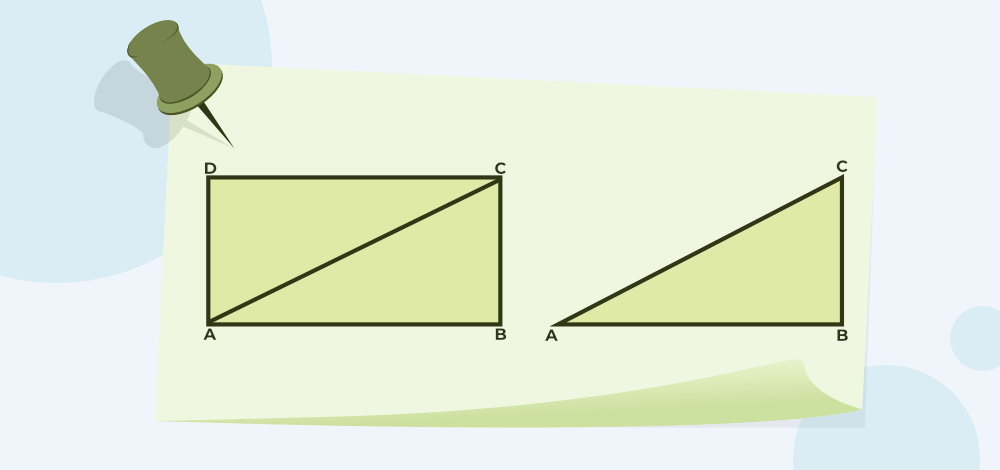GFG App
Open AppBrowser
Continue

Area of rectangle is the region covered inside the boundaries of the rectangle. A rectangle is a quadrilateral in which opposite sides are equal and parallel. The diagonals of a rectangle are equal and are perpendicular bisectors to each other. All the angles of the rectangles are 90°. A rectangle is similar to a parallelogram. We can say that all the rectangles are parallelograms but its converse is not true.

## What is the Area of a Rectangle?

DefinitionThe area of a Rectangle is the region occupied by a rectangle within its four sides or boundaries.

The area of a rectangle is the space enclosed by the boundaries of the rectangle. In general, the area of any shape is the space enclosed by the geometrical shape. We can also say that the space enclosed by the perimeter of the rectangle is the area of a rectangle. The perimeter of a rectangle is the sum of all the sides. For a rectangle, the area is the multiplication of its length by its breadth. The opposite sides of the rectangle are equal, and if the length and breadth are also equal, then the shape becomes a square. Therefore, every square is a rectangle. The below-given diagram shows the rectangle and its area,Area of Rectangle

## Unit of Area of Rectangle

The area of a rectangle is measured in square units and the standard unit for measuring the area of a rectangle is m2. Other units widely used for measuring the area of the rectangle are cm2, mm2, and others.

## Area of a Rectangle Formula

The formula for the area of a rectangle is the multiplication of length (L) and breadth (B).

where ,
L is the length of the rectangle
B is the breadth of the rectangle

Note:-

If the unit of length and breadth is not exact then it should be transformed into one unit. For e.g. If the length is in cm and breadth in m then both dimensions should be adjusted either to m or cm.

## How to Find the Area of a Rectangle?

The area of the rectangle is defined as the product of its length and breadth. Following are the steps that help calculate the area of the rectangle,

Step 1: Note down the dimensions of the rectangle.

Step 2: Calculate the product of the length and breadth of the rectangle.

Step 3: Write the answer in respective square units.

### Example: Find the area of a rectangle whose length is 20 inches and breadth is 50 inches.

Solution:

The formula for area of rectangle is given:

Area = L × B

Area = 20 × 50

Area = 1000 inches2

Thus, the required area is 1000 inches2

## Area of a Rectangle with Diagonal

The area of the rectangle can be found by two methods which are:

### Method 1:

We can find the value of the missing side using the Pythagoras theorem and then find the area. Let us understand this using an example.

The diagonal of the rectangle is the line joining opposite vertices. The diagonal of the rectangle is calculated using Pythagoras’s TheoremMethod 1 to find the Area of the Rectangle

The formula for the area of a rectangle is calculated by:

### Method 2:

If both the diagonals of the rectangle are given then its area can be found with the help of the area of the quadrilateral formula.

Let a rectangle ABCD have diagonals as AC and BD and their length is d1 and d2 then its area is given by,

Area of rectangle ABCD = 1/2 × d1 × d2

### Example: Find the area of a rectangle whose length of the diagonals is 10 cm and 14 cm.

Solution:

The formula for area of rectangle is,

Area = 1/2 × d1 × d2

Area = 1/2 × 10 × 14

Area = 70 cm2

Thus, the area of required rectangle is 70 cm2.

## Area of Rectangle using Perimeter

To calculate the area of a rectangle using the perimeter and one dimension follow the following steps,

Step 1: Note the perimeter and the given dimension.

Step 2: Use the perimeter formula to find the other dimension.

Step 3: Use the area of the rectangle formula and substitute the required value obtained in Step 2

Step 4: Simplify the expression and add unit to get the final answer.

The example given below explains the above concept.

### Example: Find the area of a rectangle when the perimeter is 28 cm and the breadth is 8 cm.

Solution:

Given,

Perimeter of Rectangle = 28 cm

length = 8 cm

Using Perimeter of rectangle formula,

Perimeter of rectangle = 2 (l + b)

28 = 2 (8 + b)

14 = 8 + b

b = 6 cm

Thus the breadth of rectangle is 6 cm

Area of Rectangle = l × b

= 8 × 6 = 48 cm2

Thus, the area of the Rectangle is 48 cm2

## Derivation of Area of a Rectangle

The area of a rectangle is the product of length and breadth. This can be derived by dividing the rectangle into two triangles. The triangles are equal as the base and height of the two triangles will be equal.

Let’s derive the formula for the area of a rectangle, the image given below shows that a rectangle is made by joining two equal right-angle triangles.Area of a Rectangle Formula Derivation

• Area of Rectangle = 2 (Area of Triangle)
• Area of Rectangle = 2 (1/2 × Base × Height)
• Area of Rectangle = 2 (1/2 × AB × BC)
• Area of Rectangle = AB × BC
• Area of Rectangle = Length × Breadth.

Thus, the area of the rectangle formula is derived.

## Solved Examples on Area of Rectangle

### Example 1: The length and width of a rectangle are 6 units and 3 units, respectively. Find the area of the rectangle.

Solution:

Given,

length = 6 unit

Area of rectangle =  length × breath
=  6 × 3
= 18 square units

Thus, the area of given rectangle is 18 square units

### Example 2: Find the area of a rectangle whose height is 10 cm and width is 2 cm.

Solution:

Given,

Height = 10 cm
Width = 2 cm

Area of Rectangle = width × height

Area of Rectangle = 10 × 2 = 20

Therefore, the area of the rectangle is 20 cm2.

### Example 3: A rectangular panelhas a width of 150 mm and a height of 99 mm. Find the area of this panel.

Solution:

Given,

Height = 99 mm
= 9.9 cm

Width = 150 mm = 15 cm

Area of Rectangle = width × height

Area of Rectangle = 15 × 9.9

Therefore, the area of the rectangle = 148.5 cm2.

### Example 4: The height of a rectangular net is seen to be 20 cm. Its area is seen to be 260 cm2. Find the width of the provided net.

Solution:

Given,

Height = 20 cm
Area = 260 cm2

Area of Rectangle = width × height

Therefore,

width = Area / height

width = 260/20

width = 13 cm

Thus, the width of the rectangle is 13 cm

### Example 5: The height and width of a rectangular desk are 40 m and 20 m, respectively. If a carpenter charges ₹ 2 per m2 for his work, how much would it cost to make the whole desk?

Solution:

Given,

Height of Desk = 40 m
Width of Desk = 20 m

Area of top of Desk = width of desk × height of desk

Area of top of Desk = 40 × 20

Area of top of Desk = 800 m2

At the cost of ₹ 2 per m2

The cost for making top of the desk is 800 × 2 = ₹ 1600

### Example 6: A wall whose length and width are 60 m and 40 m respectively needs to be painted. Find the quantity of the paint required if 1 litre of paint can paint 400 m2 of the wall.

Solution:

Given,

Length of wall = 60 m

Width of wall = 40 m

Area of wall = width × length

Area of wall = 60 × 40

Area of wall = 2400 m2

Paint required for 400 m2 of wall = 1 litre   (given)

Paint required for 2400 m2 of wall = 2400 / 400 × 1 = 6 litre.

Thus, the paint required to paint the wall is 6 litre.

### Q7: If the length and breadth of the rectangular glass slab are 6 m and 4 m respectively. Then finds its area.

Solution:

Length of the rectangular slab is 6 m

Breadth of the rectangular slab is 4 m

Area of rectangular slab = length × breadth

= 6 × 4
= 24 m2

Here, the area is measured in m2.

Related Articles:

## FAQs on Area of Rectangle

### Q1: What is the formula for the area of a rectangle?

Area (A) of rectangle formula is the product of the length and breadth. It can be defined as the space occupied by its boundaries.

### Q2: What is the unit of area of a rectangle?

The unit of the area of rectangle are meter2, centimeter2, inches2, etc. In general, it is unit2.

### Q3: What is the perimeter of a rectangle?

The perimeter of rectangle is the sum of the length of all its boundaries. The formula for perimeter of rectangle is given as;

P = 2 (Length + Breadth)

### Q4: How to find the area of a rectangle?

For a rectangle whose length is l and breadth is b then its area can be calculated by using the formula,

Area = l × b

### Q5: How to find the area of a rectangle with a diagonal?

The area of the rectangle when its diagonal is given is calculated using the formula,

Area = 1/2 × d1 × d2

where
d1 is the first diagonal.
d2 is the second diagonal.

### Q6: How to find the area of a rectangle when a perimeter is given?

The area of a rectangle when its perimeter and any one side is given can be calculated by,

Step 1: Use the perimeter of the rectangle formula to find the relation between length (l) and breadth (b).

Step 2: One dimension is already given. Use the relation to find the other dimension.

Step 3: When both dimensions are known use the area formula to find the area.

### Q7: How to calculate the area of a quadrilateral with 4 different sides?

The area of a quadrilateral with all fours sides different and both diagonal given are,

Area = 1/2 × d1 × d2

where
d1 is the first diagonal.
d2 is the second diagonal.